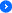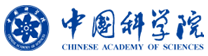数学院综合集成与知识科学研究组Location :home > MCS Seminar > Latest Seminar > Seminar List
A Study on Scale Free Social Network Evol...

Inspired by real world phenomena, this talk presents a new power law evolutional network model with degree exponent β<2. We use combinatorial probabilistic methods to examine the evolution of on line social networks. In our model, each time stamp the number of links among a new added node and old ones follows Poisson distribution with parameter λ and selection
probability
p. We derive exact analytical relationship between the exponent of the power law β and the parameter λ, p. Through the computer simulations, we verify the correctness of the exponent β analytical solutions. Both theoretical solutions and simulation results show that the presented network evolution model with randomly growing edges conforms to degree exponent
β<2 and bending phenomena observed in real world network.CAS,Research Group of Meta-Synthesis and Knowledge Science